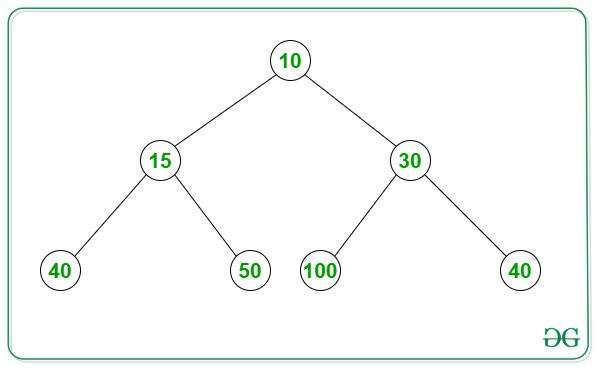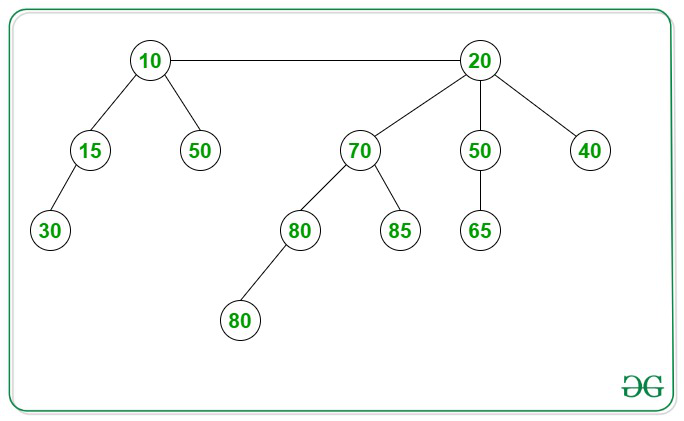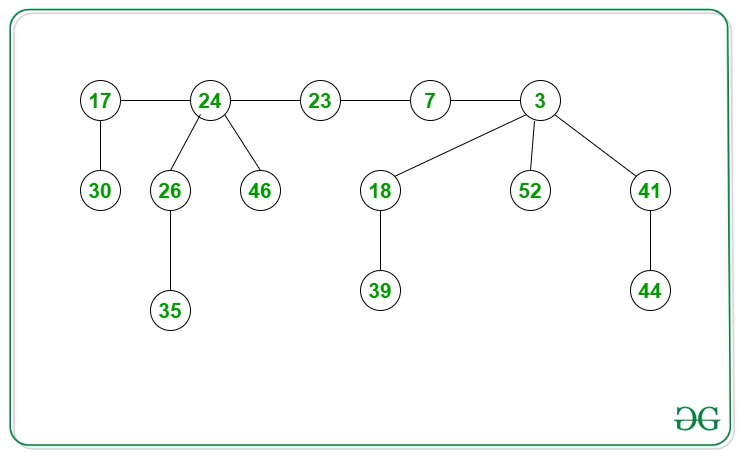Open in App
Not now

# Difference between Binary Heap, Binomial Heap and Fibonacci Heap

• Difficulty Level : Medium
• Last Updated : 03 Nov, 2020

Binary Heap:
A Binary Heap is a Binary Tree with following properties.

1. It’s a complete binary tree i.e., all levels are completely filled except possibly the last level and the last level has all keys as left as possible. This property of Binary Heap makes them suitable to be stored in an array.
2. A Binary Heap is either Min Heap or Max Heap. In a Min Binary Heap, the key at root must be minimum among all keys present in Binary Heap. The same property must be recursively true for all nodes in Binary Tree. Max Binary Heap is similar to Min Heap.

Example of Min-Heap:Binomial Heap:
A Binomial Heap is a collection of Binomial Tree where each Binomial Tree follows the Min-Heap property and there can be at most one Binomial Tree of any degree.

Example of Binomial Heap:The key difference between a Binary Heap and a Binomial Heap is how the heaps are structured. In a Binary Heap, the heap is a single tree, which is a complete binary tree. In a Binomial Heap, the heap is a collection of smaller trees (that is, a forest of trees), each of which is a binomial tree. A complete binary tree can be built to hold any number of elements, but the number of elements in a binomial tree of some order N is always 2*N. Consequently, one complete binary tree is needed to back a Binary Heap, but we may need multiple binomial trees to back a Binomial Heap.

Fibonacci Heap:
Like Binomial Heap, Fibonacci Heap is a collection of trees with Min-Heap or Max-Heap property. In Fibonacci Heap, trees can have any shape even all trees can be single nodes (This is unlike Binomial Heap where every tree has to be a Binomial Tree). Fibonacci Heap maintains a pointer to a minimum value (which is the root of a tree). All tree roots are connected using a circular doubly linked list, so all of them can be accessed using a single ‘min’ pointer.

Example of Fibonacci Heap:The difference in time complexities of various operations associated with Binary heap, Binomial heap, and Fibonacci heaps are mentioned in the following table.

My Personal Notes arrow_drop_up
Related Articles